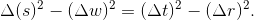iSoul In the beginning is reality.

# Invariant intervals

The spacetime interval is invariant over the Lorentz transformation (LT). The following is a proof of this for the inverse LT with length space axes x, y, and, z; temporal axis t (time line), velocity v, and maximum velocity c, along with β = v/c and γ = 1/√(1 − β²):The invariant interval is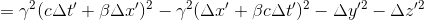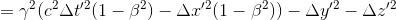Expand the squares and cancel the middle terms to get: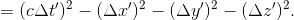The timespace interval is invariant over the dual Lorentz transformation (DLT). The following is a proof of this for the inverse DLT that follows with temporal axes x, y, and, z; stantial axis r (stanceline), lenticity u, and maximum lenticity κ, along with ζ = u/κ and λ = 1/√(1 − ζ²):The invariant interval is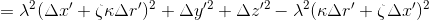Expand the squares and cancel the middle terms to get: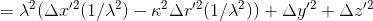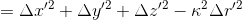Let Σ (Δri)² = (Δr)² and Σ (Δti)² = (Δt)². Then the two invariant intervals are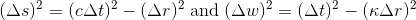These two invariant intervals are proportional if κ = 1/c:In that case, the invariant interval for proper time is the same for both 3+1 and 1+3 dimensions. However, 3D proper time is limited to space-like intervals, while 3D proper distance is limited to time-like intervals.

If c = κ = 1 (without regard to units), then these invariant intervals are nominally the same: